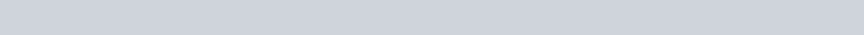Miami Dade College Specific Heat Capacity of Nickel Coins Lab Report

Experiment – Specific Heat Capacity of Nickel Coins

Directions: To complete the experiment, read the laboratory experiment in the manual, watch the video below, and use the simulated data to complete the data table below.

The experimental report must be submitted to the appropriate Blackboard assignment folder.

Reading Assignment: Pages 68 – 74 Lab Manual

Specific Heat Capacity of Nickel Coins

 Experimental Videos: Specific HeatCapacity of Nickel Coins https://youtu.be/8gHFaL2990U  The video shows a similar experiment. Do not use the information in the video. Use the simulated data.

Post Lab Questions

1. Answer questions 1, 4 and 5 from lab manual.

Simulated Data:

Complete the data table.

Specific Heat Capacity of Nickel Coins

Specific Heat Capacity of Nickel Coins Data Sheet

 Trial 1 Trial 2 Trial 3 Mass of nickel coins (g) 99.419 99.419 99.419 Volume of water used (mL) 70 70 70 Mass of water used (g) 67.951 68.752 68.819 Initial Temperature of nickel Coins (Nickel Coins temp. before transfer) (°C) 94 90 92 Initial Temperature of water (water temp. before transfer) (°C) 19 18.5 18 Final Temperature of Nickel Coins (°C) 29 27 26 Final temperature of water (°C) 29 27 26 ΔT of nickel coins (°C) ΔT of water (°C) Heat absorbed by water (J) Heat lost by nickel coins (J) Specific heat of nickel coins (J/g °C)

 Time (To construct the graph) Temperatures (℃) Trial 1 Trial 2 Trial 3 0 19 18.5 18 10 19 18.5 18 20 19 18.5 18 30 19 18.5 18 40 19 18.5 18 50 26 25 24.5 60 28.8 27 26 70 29 27 26 80 29 27 26 90 29 27 26 100 29 27 25

 Overall Results Average specific heat of Nickel Coins (J/g °C) Standard Deviation RSD

Determination of the S ecific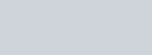aci Of Nickel Coins usin a Coffee Cu Calorimeter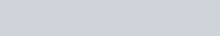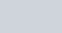specific capacity or specific heat is the amount of heat required to raise the temperature of 1 g ofa substance IOC, therefore it is a ratio ofheat to amount, and is an intcnsive property. “Ille hcnt capacity is the amount of heat that any mass of a substance can absorb in order to raise its temperature IOC; it is an cxtcnsivc propcriy.

In order to determine the specific heat capacity wc nccd to mcasurc out a mass ofthc SUbstance, in this casc nickel coins, and measure how much heat is absorbed for the mass that has been weighed on a per gram basis. amount ofhcat encrgy (J or cal) Spcciflc Ilcat = mass (g) X AT(OC)

From the units of specific heat, it should be apparent that the amount of heat energy (q):

# q = mSAT

is equal to the product of the mass, specific heat and temperature change (final temperature — initial temperature). In ordcr to determine the specific heat of nickel coins, you will measure the amount of heat transferred by the nickel coins to a known mass of water because the heat energy lost from the nickels = the heat energy gained by the water (mass x Specific Heat X coins = —(mass x Specific Heat x AT)water

or more simply as: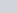mcoln X Scofn X ATcoIn = —mwater X Swater X ATwater

where m is mass, S is specific heat, and AT is the change in temperature. The specific heat Of

1 cal 4.184 J water is — ¯ CC

Safety Precautions

You are using common materials here that do not pose a known health risk. You are heating metals up to elevated temperatures, so you should be careful not to bum yourself — you should not allow hot metal to come into contact with your skin at any time. If you do, immediately put the area of skin under large volumes Of cold, running water to dissipate the heat and minimize the potential for a bum and blistering. As with all laboratory experiments, wear safety glasses at

REMEMBER TO BRING 60 NICKEL COINS To THE LABORATORY.

Illis document was prepared by D. Finncran, J. Tierney.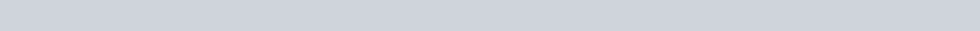68Procedure

1. Obtain the mass of20 nickels using the balance.
2. Place the nickels into a test tube.
3. Place a thermometer into the test tube on top of nickels, using the last nickel (the 20th) to keep the thermometer from touching the sides of the tube, shown in Figure 1.
4. The test tube should be clamped and immersed in a beaker of water over a stirrer/hotplate— Figure 2. Ensure the level Of Water is ABOVE all of the nickel coins in the test tube. The water should be heated to boiling.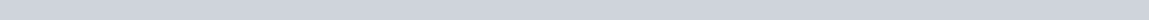Figure 2Fi

rel

1. As the water is set to boil, obtain a Styrofoam cup with lid. Record the mass of the empty cup and lid.
2. Accurately measure somewhere between 65 and 75 mL of water, using a 100 mL graduated cylinder. Record the actual volume.
3. Add the water to the Styrofoam cup. Record the mass ofthe cup, lid, and water.
4. Determine the mass ofwater by difference.
5. Place a temperature probe through the lid into the water in the Styrofoam cup.

This document was prepared by D. Finneran, J. Tiemey.

69

1. When the temperature Of the nickels reaches approximately 850C, start recording the temperature of the water in the Styrofoam cup. The temperature of the water in the Styrofoam cup should NOT change. This will be the initial temperature of the water.

I l. When the temperature of the nickels on the hotplate reaches approximately or above, you should get ready to transfer them to the Styrofoam cup. This operation has to be done quickly and smoothly in order to minimize heat loss in the transfer process. greater the temperature ofthe nickel coins, the better.

12. Carefully undo the clamp holder holding the clamp to the test tube. You are going to hold the clamp so that you do not burn yourself with the hot test tube. When you are ready to transfer the nickels, first RECORD the temperature of the nickel coins. This will be the initial temperature of the nickel coins. Then quickly remove the thermometer from the test tube and quickly remove the test tube from the hot water and place the nickels into the ‘coffee cup calorimeter.’ When you are adding the nickels to the ‘coffee cup calorimeter,’ you should ensure that the temperature probe does not come out of the water in the cup — see Figure 4.

Figure 413.

As

soon

as

all

the

NSTRUCTOR

DEMONSTRATE) and continue recording the temperature of the water for the next five

14, At the end offive minutes, stop the data collection.

1. save and export the data – your instructor will demonstrate how to do this.

This document was prepared by D. Finneran, J. Tiemey.70

1. Repeat this process two more times. Hint Have all three trials of coins warming up at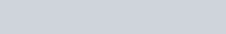Calculations

1. Using your saved create a scatter plot where Time is the independent variable (xtuxis) and Temperature is the dependent variable (y-axis). You will not see the mæximum temperature from the data but will have to determine the maximum temperature by extrapolation.

Plot of Temperature vs time

30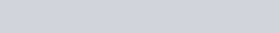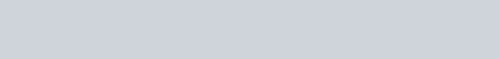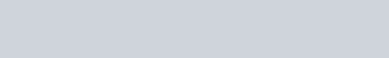inidal temp =18.50C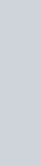28

26

24

= 22

20

18 o 100 200 300 400 500 600 700 BOO 900Time (secs)

1.Determine the final temperature.
2. Determine the heat energy gained by the water (qwater)•.

qwater = mwater X Swater X ATwaterwhere m is mass, S is specific heat, and AT is the change in temperature. The specific

1 cal 4.184 J heat of water is —cc ¯ cc

1. Determine the heat energy lost by the nickel coins (qcoin):

qcoin = ¯qwater

where m is mass, 1 cal S is 4.184 specific J heat, and AT is the change in temperature. The specificheat of water is —goc ¯ goc

1. Determine the specific heat of the nickel coin (Scoin) by rearranging the following

equation. qcoin = mcoin X Scoin X ATcoin

This document was prepared by D. Finneran, J. Tierney.

71

where m is mms•s, S is specific heat, and AT is the chnnge Jn telnpertt(urc,

1. Repeat these calculations for your other two trials.
2. Determine the average specific bent cnpnclty, Ntnndnrd dcvln(ion stnd RSJ). provide this information to the class by posting on (lic Course Mmjngement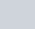(ANGEL, Blackboard, etc.) or othenvlsc Instruclcd wltJ11n 48 hourx or compJetJon of the laboratory experintent.

This document was prepared by D. Finneran, J. Tierney.

72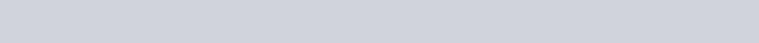Specific Heat Capacity of Nickel Coins Data SheetName

This document was prepared by D. Finneran, J. Tierney. 73

Specific Heat Capacity of Nickel Coins Post Lab Questions

Name Partner’s Name

Please type your answers to the following questions on as many pages as needed. Non-typed answers will not be accepted

1. The density of water is oftentimes assumed to be 1 g ml.-l. Does the data collected validate or invalidate this assumption? Explain.
2. How does the specific heat capacity of the nickel coin you determined compare to the class average? Explain.1. How does your precision for the specific heat capacity of a nickel coin compare to the class? Explain.
2. What is the specific heat capacity of elemental nickel? Remember to cite the reference used.
3. What is the specific heat capacity of elemental copper? Remember to cite the reference
4. What is a nickel coin actually composed of and in what proportion? Remember to cite the reference used.
5.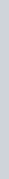What is the actual specific heat capacity of a nickel coin? Show appropriate calculations.
6. How does the average specific heat capacity of the nickel coin you determined experimentally compare to the actual specific heat capacity of a nickel coin you calculated in question 7? Explain.
7. Why do you need to determine the final temperature by extrapolation?
8. For the purposes of this experiment, it is assumed that the coffee cup does NOT absorb any heat. How could this assumption be tested?
9. How could you improve this laboratory experiment?This document was prepared by D. Finneran, J. Tiemey.

74